# 图解ConcurrentHashMap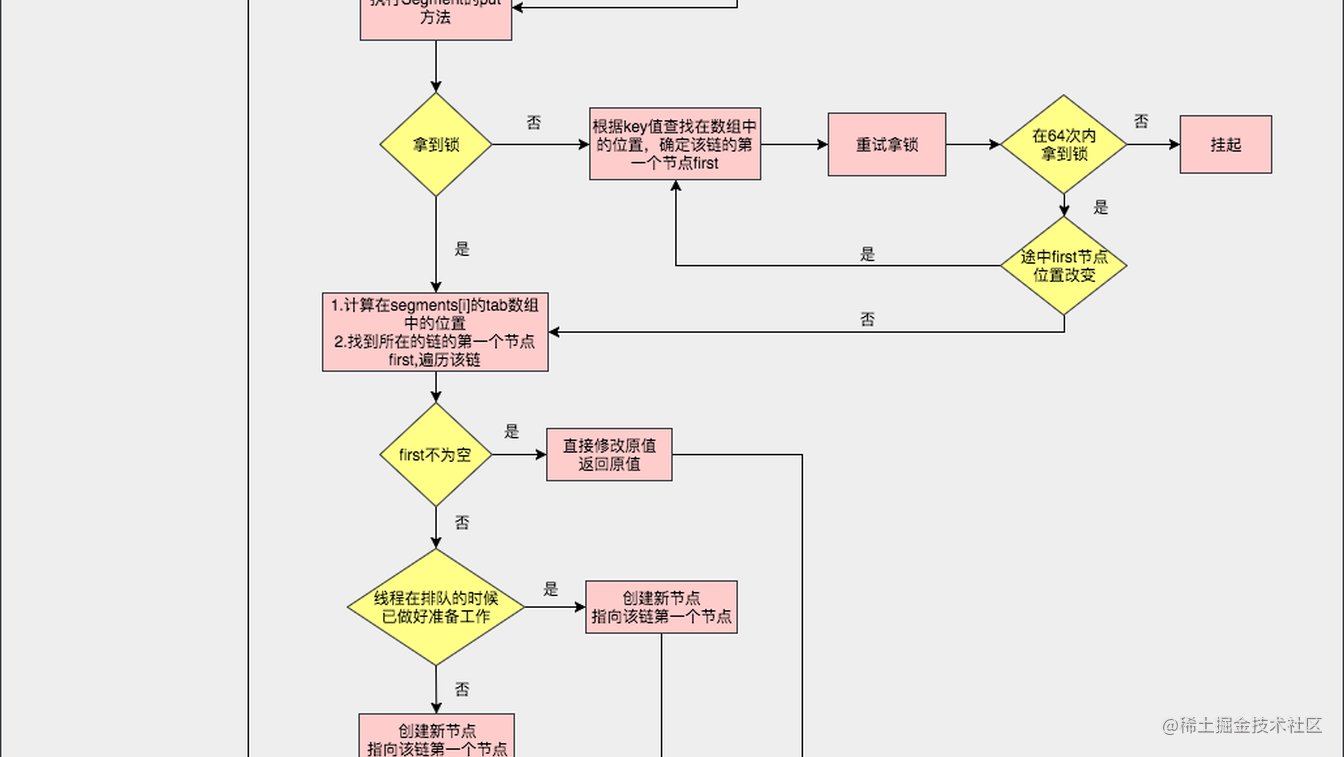### ConcurrentHashMap是什么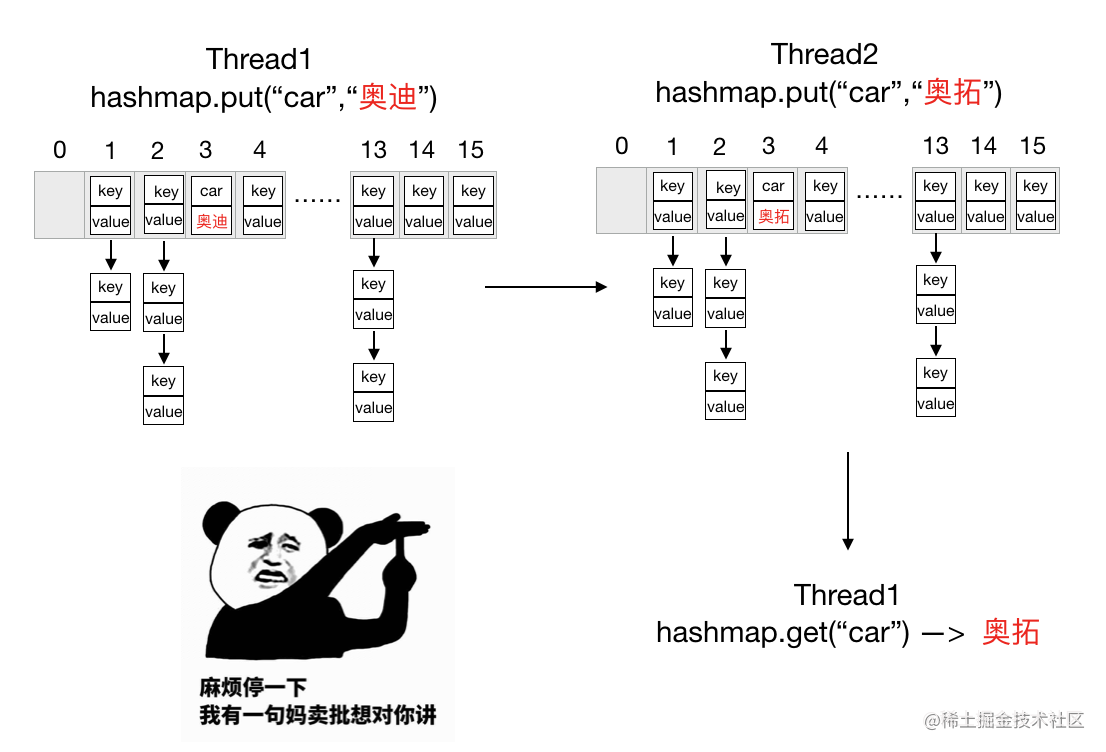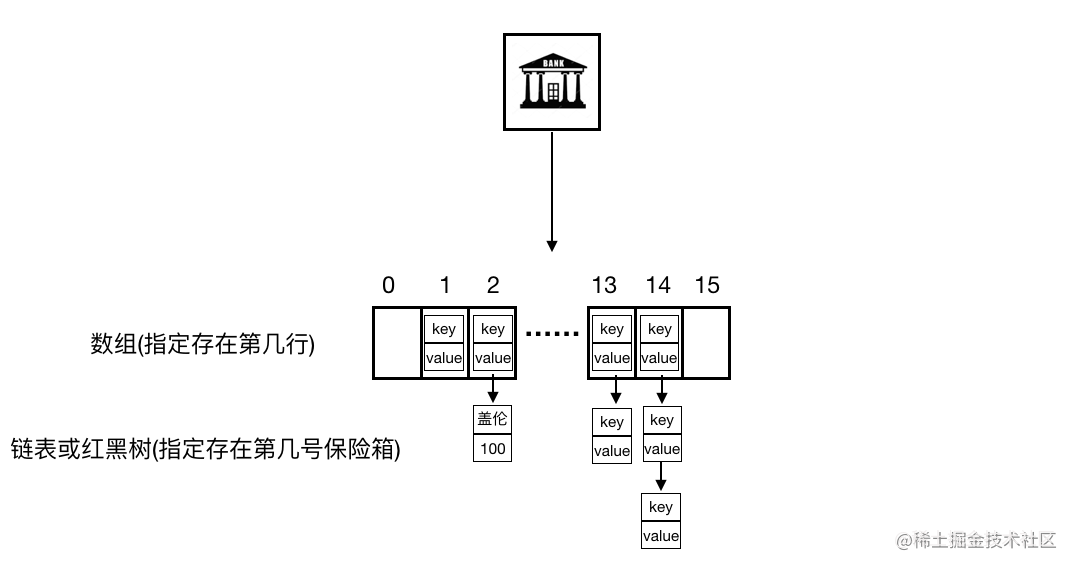• HashMap牌银行：我们的服务宗旨是不用排队，同一时间多人都有机会修改保险柜里的东西，你以为你存的是美元？取出来的其实是日元，破产就在一瞬间，刺不刺激。
• HashTable牌银行：我们的服务宗旨是要排队，同一时间只有一个人有机会修改保险柜里的东西，其余的人只能看不能动手改，保你存的是美元取得还是美元。什么？你说如果那人在里面睡着了不出来怎么办？不要着急，来，坐下来打会麻将等他出来。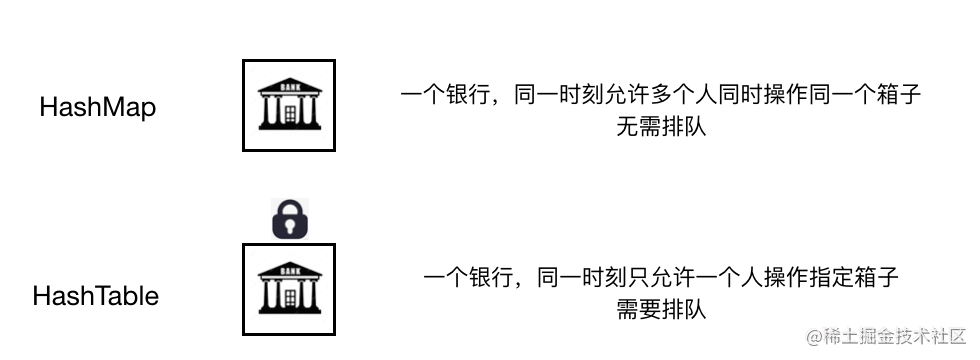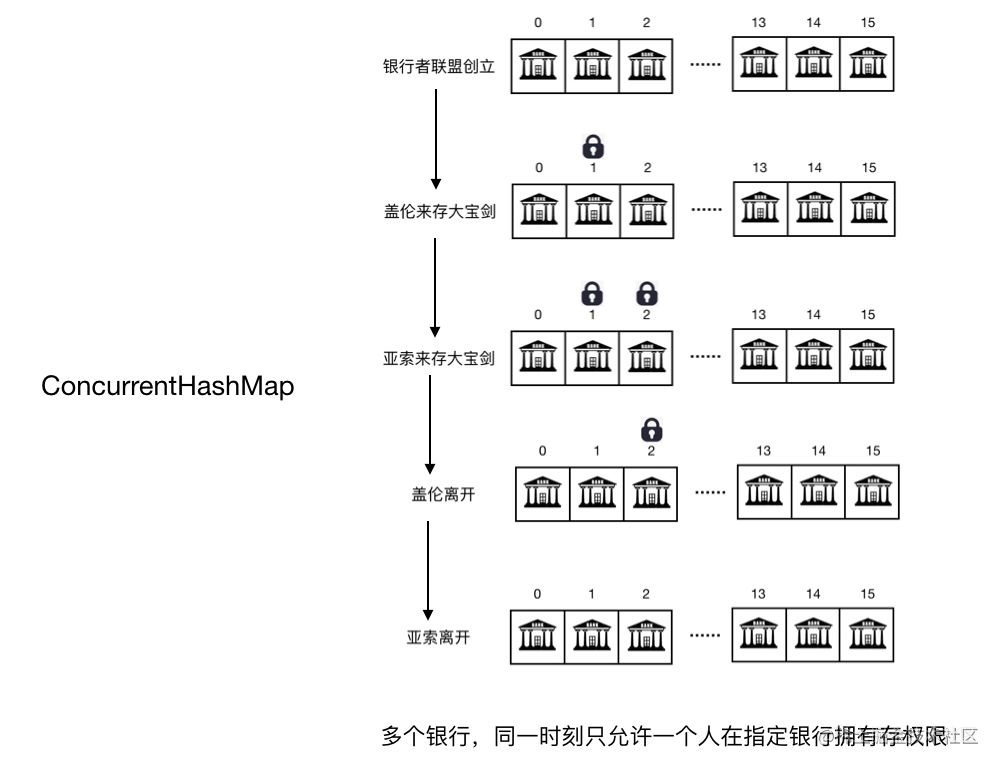• 多个银行组成的「银行者联盟」
• 当有人来办理业务时，「银行者联盟」需要确定这个人去哪个银行
• 当此人去到指定银行办理业务后，该银行上锁，其他人不能同时执行修改操作，直到此人离开后解锁

1.成立「银行者联盟」时初识银行数是多少？怎么设计合理？

2.当有人来办理业务的时候，「银行者联盟」怎么确定此人去哪个银行？

3.「银行者联盟」怎么保证同一时间不会有两个人在同一个银行拥有存权限？

### 源码分析

#### Java7 源码分析

``````//默认的数组大小16(HashMap里的那个数组)
static final int DEFAULT_INITIAL_CAPACITY = 16;

//扩容因子0.75
static final float DEFAULT_LOAD_FACTOR = 0.75f;

//ConcurrentHashMap中的数组
final Segment<K,V>[] segments

//默认并发标准16
static final int DEFAULT_CONCURRENCY_LEVEL = 16;

//Segment是ReentrantLock子类，因此拥有锁的操作
static final class Segment<K,V> extends ReentrantLock implements Serializable {
//HashMap的那一套，分别是数组、键值对数量、阈值、负载因子
transient volatile HashEntry<K,V>[] table;
transient int count;
transient int threshold;

Segment(float lf, int threshold, HashEntry<K,V>[] tab) {
this.threshold = threshold;
this.table = tab;
}
}

//换了马甲还是认识你！！！HashEntry对象，存key、value、hash值以及下一个节点
static final class HashEntry<K,V> {
final int hash;
final K key;
volatile V value;
volatile HashEntry<K,V> next;
}
//segment中HashEntry[]数组最小长度
static final int MIN_SEGMENT_TABLE_CAPACITY = 2;

//用于定位在segments数组中的位置，下面介绍
final int segmentShift;

``````ConcurrentHashMap concurrentHashMap = new ConcurrentHashMap();

``````    public ConcurrentHashMap() {
}

public ConcurrentHashMap(int initialCapacity,
if (!(loadFactor > 0) || initialCapacity < 0 || concurrencyLevel <= 0)
throw new IllegalArgumentException();
if (concurrencyLevel > MAX_SEGMENTS)
concurrencyLevel = MAX_SEGMENTS;
// Find power-of-two sizes best matching arguments
//步骤① start
int sshift = 0;
int ssize = 1;
while (ssize < concurrencyLevel) {
++sshift;
ssize <<= 1;
}
this.segmentShift = 32 - sshift;
//步骤① end
//步骤② start
if (initialCapacity > MAXIMUM_CAPACITY)
initialCapacity = MAXIMUM_CAPACITY;
int c = initialCapacity / ssize;
if (c * ssize < initialCapacity)
++c;
int cap = MIN_SEGMENT_TABLE_CAPACITY;
while (cap < c)
cap <<= 1;
//步骤② end
// create segments and segments
//步骤③ start
Segment<K,V> s0 =
(HashEntry<K,V>[])new HashEntry[cap]);
Segment<K,V>[] ss = (Segment<K,V>[])new Segment[ssize];
UNSAFE.putOrderedObject(ss, SBASE, s0); // ordered write of segments
this.segments = ss;
//步骤③ end
}

• 步骤① concurrencyLevel = 16 ，可以计算出 sshift = 4，ssize = 16，segmentShift = 28，segmentMask = 15；
• 步骤② c = 16/16 = 1，cap = 2；
• 步骤③有句注释，创建 Segment 数组 segments 并初始化 segments  ，所以 s0 初始化后数组长度为2，负载因子0.75，阈值为1；再看这里的ss的初始化(重点，圈起来要考！！！)， ssize 此时为16，所以默认数组长度16，给人一种感觉正好和我们传的 concurrencyLevel 一样？看下下面的例子

ssize = 1，concurrencyLevel = 10 ssize = 1，concurrencyLevel = 8
ssize <<= 1 —> 2<10 满足 ssize <<= 1 —> 2<10 满足
ssize <<= 1 —> 4<10 满足 ssize <<= 1 —> 4<10 满足
ssize <<= 1 —> 8<10 满足 ssize <<= 1 —> 8<10 不满足 ssize = 8
ssize <<= 1 —> 16<10 不满足 ssize = 16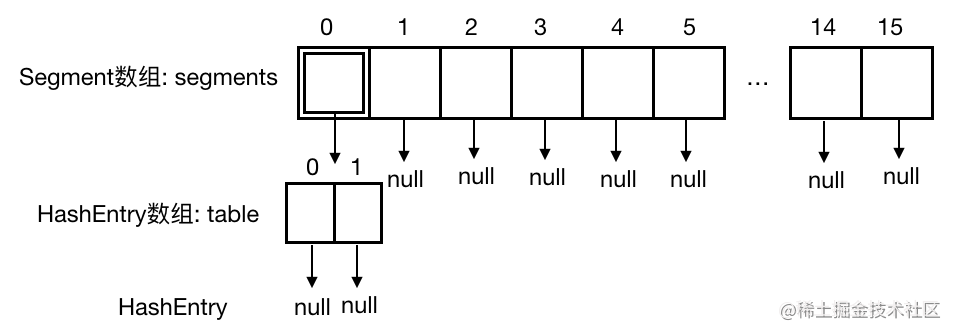``````    public V put(K key, V value) {
Segment<K,V> s;
//步骤①注意valus不能为空！！！
if (value == null)
throw new NullPointerException();
//根据key计算hash值，key也不能为null，否则hash(key)报空指针
int hash = hash(key);
//步骤②派上用场了，根据hash值计算在segments数组中的位置
int j = (hash >>> segmentShift) & segmentMask;
//步骤③查看当前数组中指定位置Segment是否为空
//若为空，先创建初始化Segment再put值，不为空，直接put值。
if ((s = (Segment<K,V>)UNSAFE.getObject          // nonvolatile; recheck
(segments, (j << SSHIFT) + SBASE)) == null) //  in ensureSegment
s = ensureSegment(j);
return s.put(key, hash, value, false);
}

``````private Segment<K,V> ensureSegment(int k) {
//获取segments
final Segment<K,V>[] ss = this.segments;
long u = (k << SSHIFT) + SBASE; // raw offset
Segment<K,V> seg;
if ((seg = (Segment<K,V>)UNSAFE.getObjectVolatile(ss, u)) == null) {
//拷贝一份和segment 0一样的segment
Segment<K,V> proto = ss; // use segment 0 as prototype
//大小和segment 0一致，为2
int cap = proto.table.length;
//负载因子和segment 0一致，为0.75
//阈值和segment 0一致，为1
int threshold = (int)(cap * lf);
//根据大小创建HashEntry数组tab
HashEntry<K,V>[] tab = (HashEntry<K,V>[])new HashEntry[cap];
//再次检查
if ((seg = (Segment<K,V>)UNSAFE.getObjectVolatile(ss, u))
== null) { // recheck
根据已有属性创建指定位置的Segment
Segment<K,V> s = new Segment<K,V>(lf, threshold, tab);
while ((seg = (Segment<K,V>)UNSAFE.getObjectVolatile(ss, u))
== null) {
if (UNSAFE.compareAndSwapObject(ss, u, null, seg = s))
break;
}
}
}
return seg;
}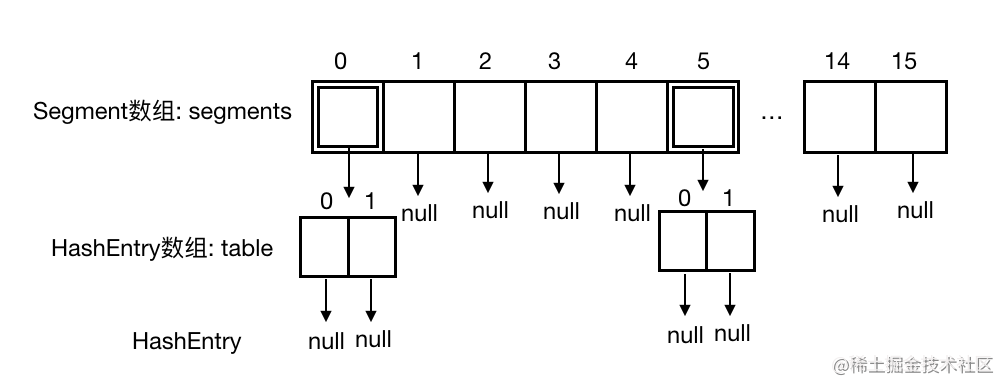``````        final V put(K key, int hash, V value, boolean onlyIfAbsent) {
//步骤① start
HashEntry<K,V> node = tryLock() ? null :
scanAndLockForPut(key, hash, value);
//步骤① end
V oldValue;
try {
//步骤② start
//获取Segment中的HashEntry[]
HashEntry<K,V>[] tab = table;
//算出在HashEntry[]中的位置
int index = (tab.length - 1) & hash;
//找到HashEntry[]中的指定位置的第一个节点
HashEntry<K,V> first = entryAt(tab, index);
for (HashEntry<K,V> e = first;;) {
//如果不为空，遍历这条链
if (e != null) {
K k;
//情况① 之前已存过，则替换原值
if ((k = e.key) == key ||
(e.hash == hash && key.equals(k))) {
oldValue = e.value;
if (!onlyIfAbsent) {
e.value = value;
++modCount;
}
break;
}
e = e.next;
}
else {
//情况② 另一个线程的准备工作
if (node != null)
//链表头插入方式
node.setNext(first);
else //情况③ 该位置为空，则新建一个节点(注意这里采用链表头插入方式)
node = new HashEntry<K,V>(hash, key, value, first);
//键值对数量+1
int c = count + 1;
//如果键值对数量超过阈值
if (c > threshold && tab.length < MAXIMUM_CAPACITY)
//扩容
rehash(node);
else //未超过阈值，直接放在指定位置
setEntryAt(tab, index, node);
++modCount;
count = c;
//插入成功返回null
oldValue = null;
break;
}
}
//步骤② end
} finally {
//步骤③
//解锁
unlock();
}
//修改成功，返回原值
return oldValue;
}

• 步骤① 执行 tryLock 方法获取锁，拿到锁返回null，没拿到锁执行 scanAndLockForPut 方法；
• 步骤② 和 HashMap 里的那一套思路是一样的，不理解可以看下之前的文章介绍(情况②下面介绍)；
• 步骤③ 执行 unLock 方法解锁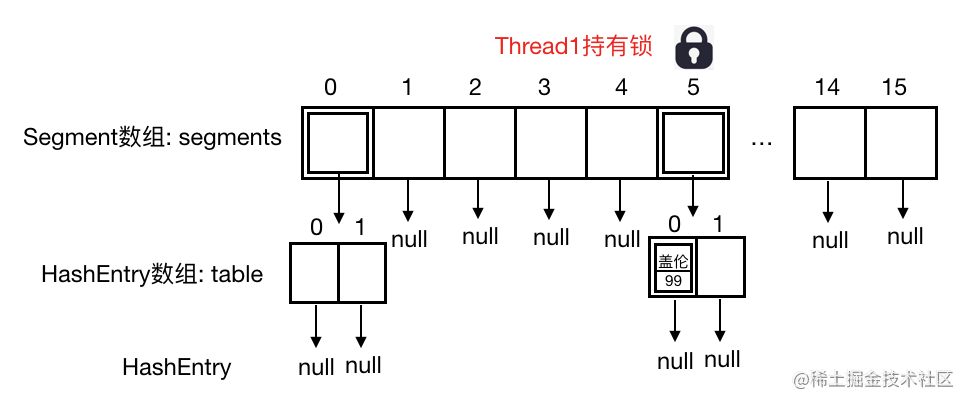``````        private HashEntry<K,V> scanAndLockForPut(K key, int hash, V value) {
//通过Segment和hash值寻找匹配的HashEntry
HashEntry<K,V> first = entryForHash(this, hash);
HashEntry<K,V> e = first;
HashEntry<K,V> node = null;
//重试次数
int retries = -1; // negative while locating node
//循环尝试获取锁
while (!tryLock()) {
HashEntry<K,V> f; // to recheck first below
//步骤①
if (retries < 0) {
//情况① 没找到，之前表中不存在
if (e == null) {
if (node == null) // speculatively create node
//新建 HashEntry 备用,retries改成0
node = new HashEntry<K,V>(hash, key, value, null);
retries = 0;
}
//情况② 找到，刚好第一个节点就是，retries改成0
else if (key.equals(e.key))
retries = 0;
//情况③ 第一个节点不是，移到下一个，retries还是-1，继续找
else
e = e.next;
}
//步骤②
//尝试了MAX_SCAN_RETRIES次还没拿到锁，简直B了dog！
else if (++retries > MAX_SCAN_RETRIES) {
//泉水挂机
lock();
break;
}
//步骤③
//在MAX_SCAN_RETRIES次过程中，key对应的entry发生了变化，则从头开始
else if ((retries & 1) == 0 &&
(f = entryForHash(this, hash)) != first) {
e = first = f; // re-traverse if entry changed
retries = -1;
}
}
return node;
}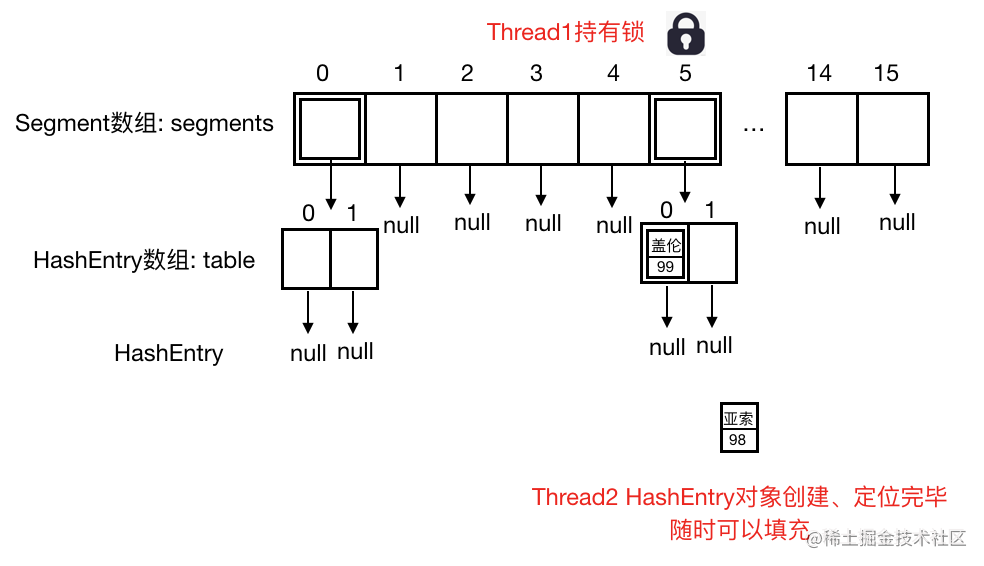``````        private void rehash(HashEntry<K,V> node) {
/*
* Reclassify nodes in each list to new table.  Because we
* are using power-of-two expansion, the elements from
* each bin must either stay at same index, or move with a
* power of two offset. We eliminate unnecessary node
* creation by catching cases where old nodes can be
* reused because their next fields won't change.
* Statistically, at the default threshold, only about
* one-sixth of them need cloning when a table
* doubles. The nodes they replace will be garbage
* collectable as soon as they are no longer referenced by
* concurrently traversing table. Entry accesses use plain
* array indexing because they are followed by volatile
* table write.
*/
//旧数组引用
HashEntry<K,V>[] oldTable = table;
//旧数组长度
int oldCapacity = oldTable.length;
//新数组长度为旧数组的2倍
int newCapacity = oldCapacity << 1;
//修改新的阈值
//创建新表
HashEntry<K,V>[] newTable =
(HashEntry<K,V>[]) new HashEntry[newCapacity];
int sizeMask = newCapacity - 1;
//遍历旧表
for (int i = 0; i < oldCapacity ; i++) {
HashEntry<K,V> e = oldTable[i];
if (e != null) {
HashEntry<K,V> next = e.next;
//确定在新表中的位置
int idx = e.hash & sizeMask;
//情况① 链表只有一个节点，指定转移到新表指定位置
if (next == null)   //  Single node on list
newTable[idx] = e;
else { // Reuse consecutive sequence at same slot
HashEntry<K,V> lastRun = e;
int lastIdx = idx;
for (HashEntry<K,V> last = next;
last != null;
last = last.next) {
//情况② 扩容前后位置发生改变
int k = last.hash & sizeMask;
if (k != lastIdx) {
lastIdx = k;
lastRun = last;
}
}
//将改变的键值对放到新表的对应位置
newTable[lastIdx] = lastRun;
// Clone remaining nodes
//情况③ 把链表中剩下的节点拷到新表中
for (HashEntry<K,V> p = e; p != lastRun; p = p.next) {
V v = p.value;
int h = p.hash;
int k = h & sizeMask;
HashEntry<K,V> n = newTable[k];
newTable[k] = new HashEntry<K,V>(h, p.key, v, n);
}
}
}
}
//添加新的节点(链表头插入方式)
node.setNext(newTable[nodeIndex]);
newTable[nodeIndex] = node;
table = newTable;
}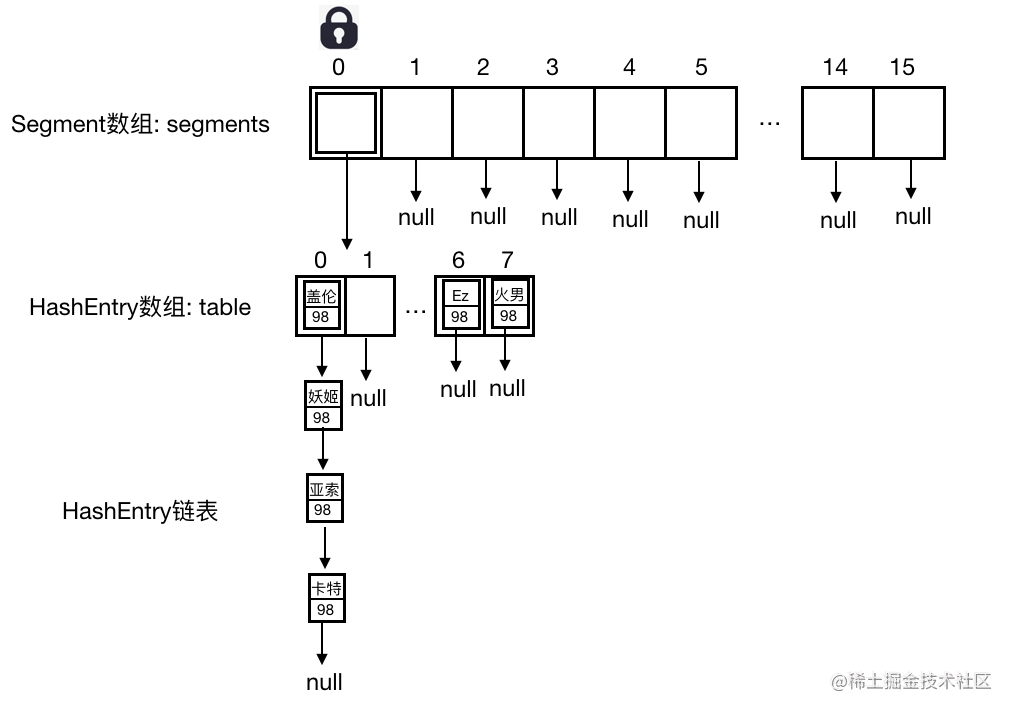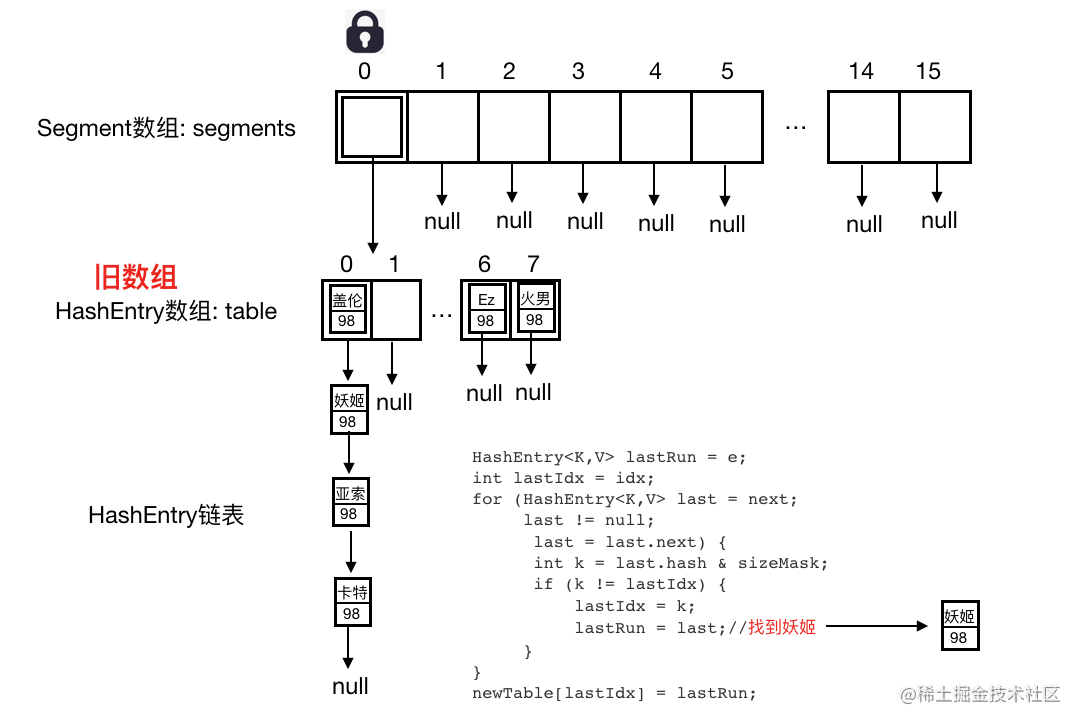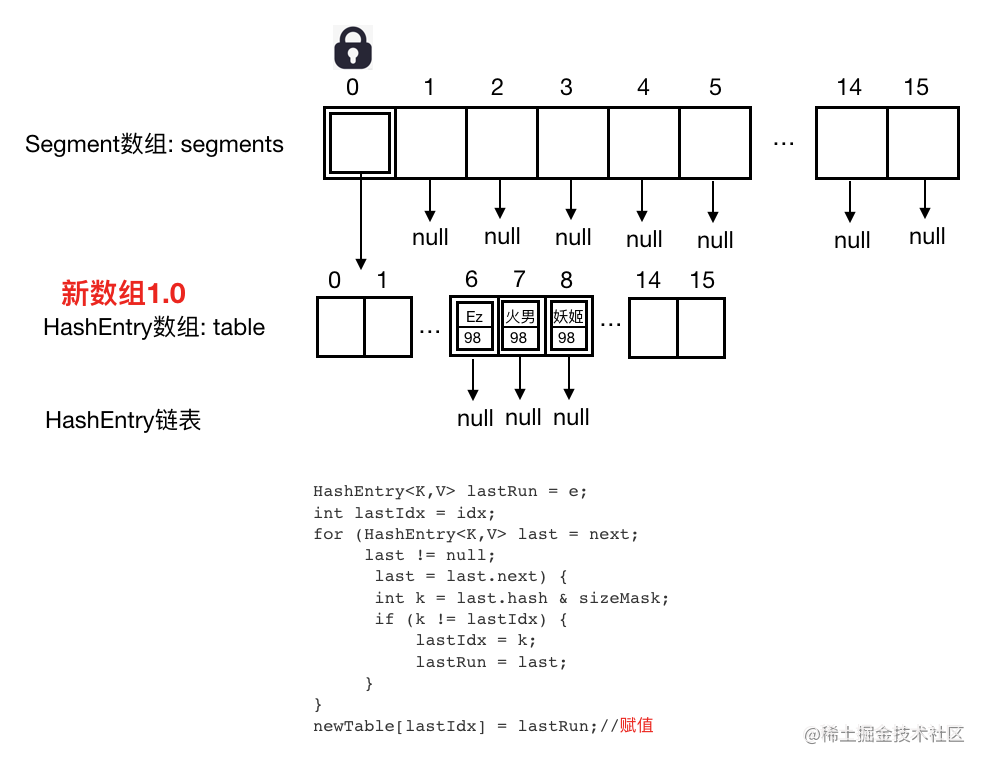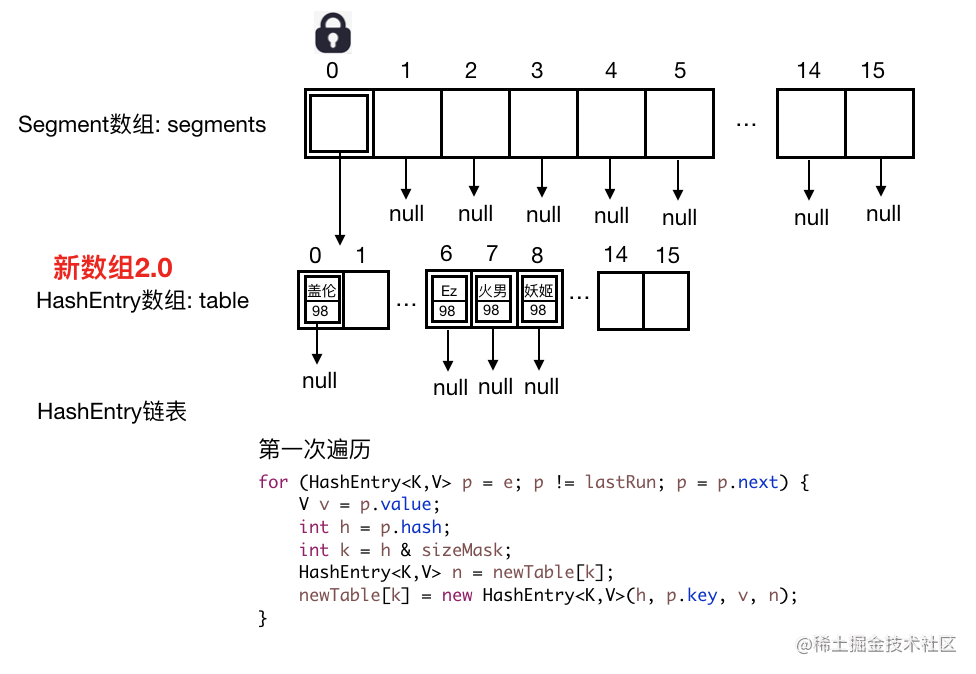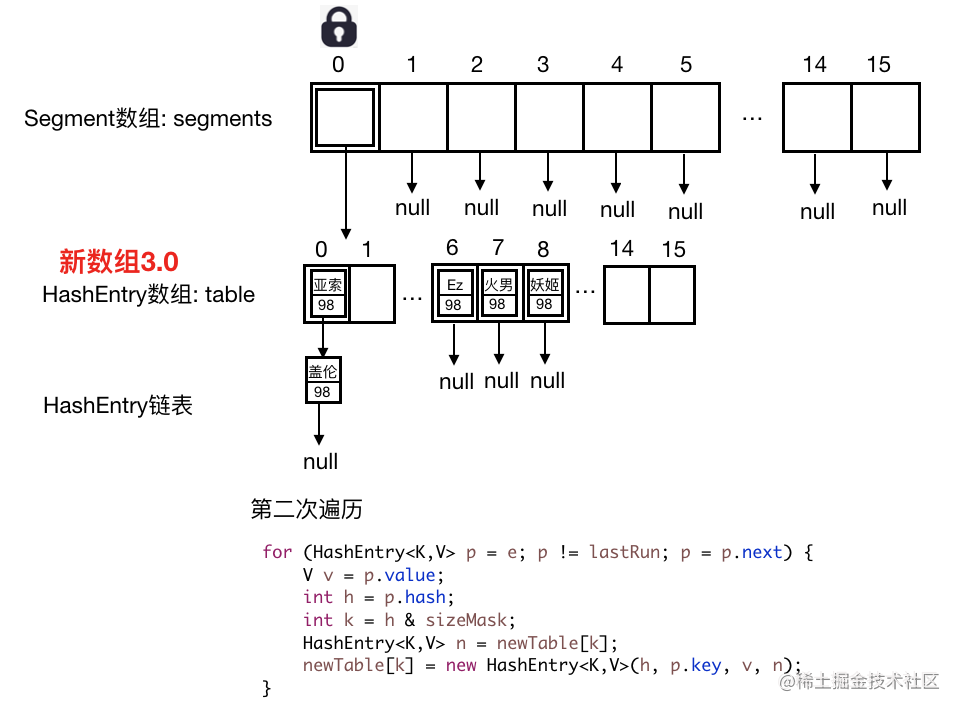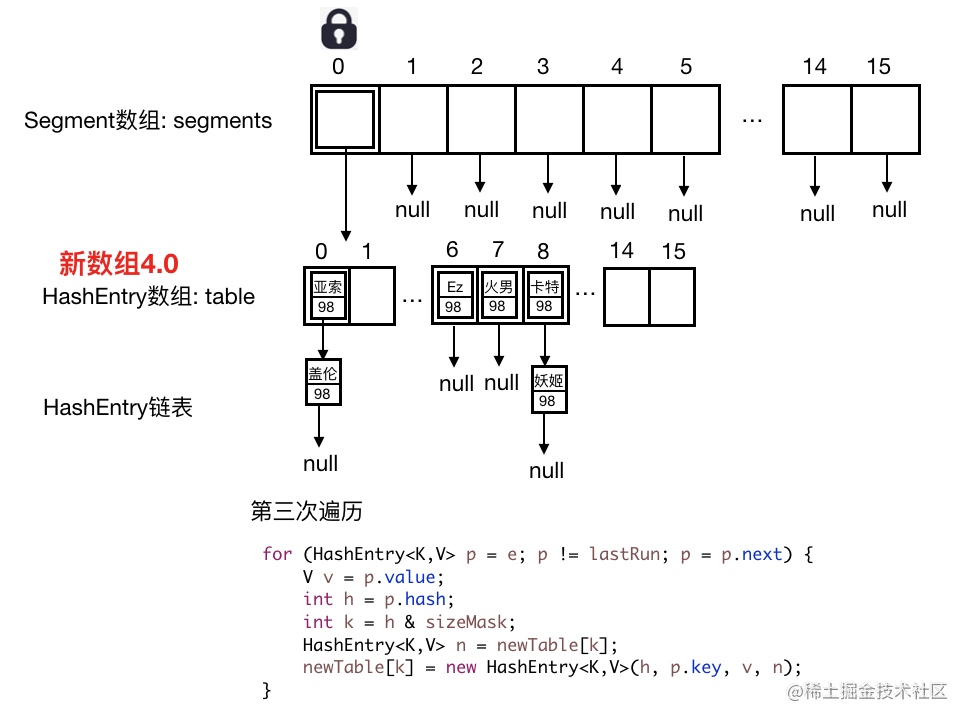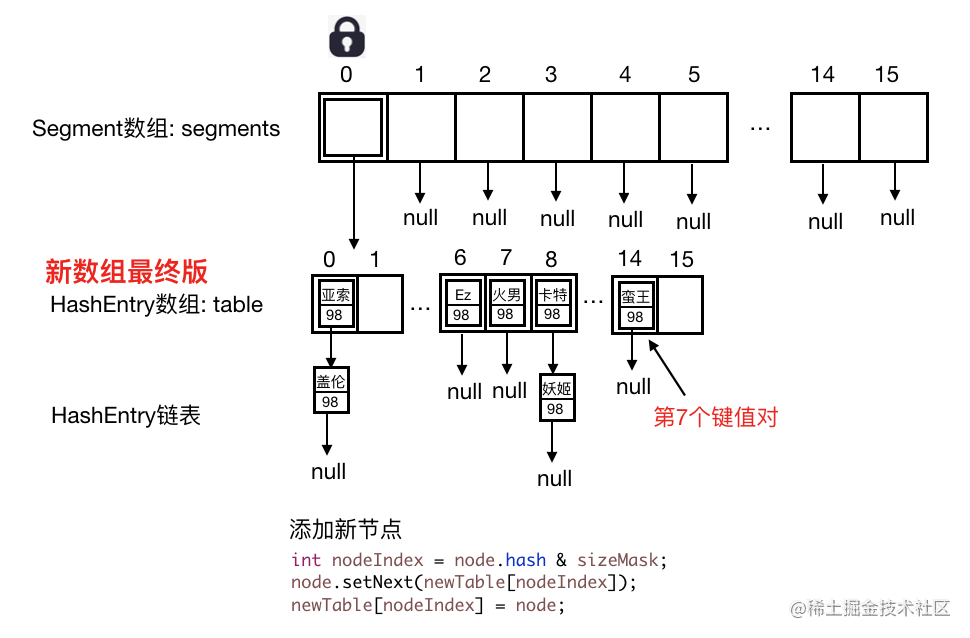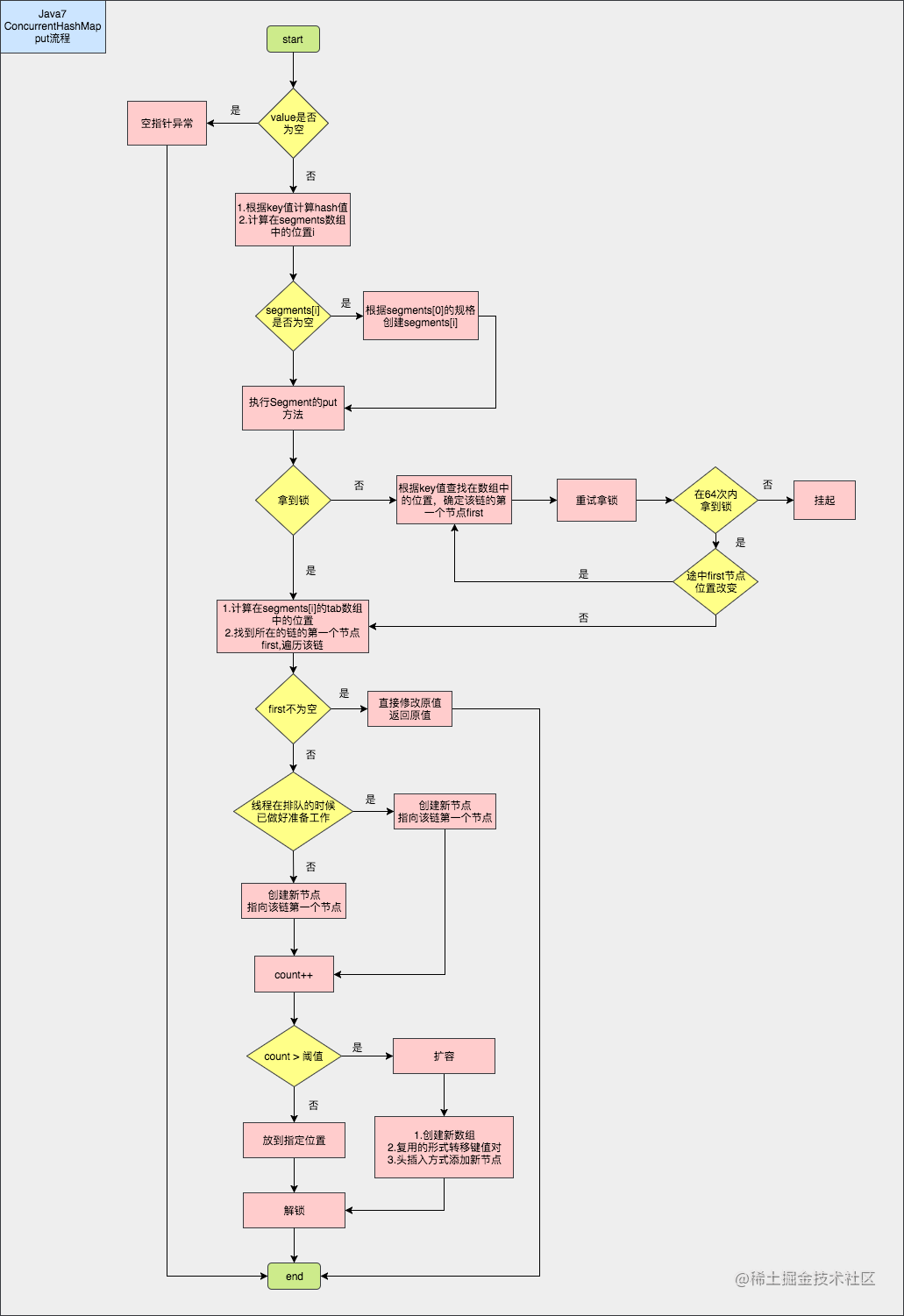#### 稍微说下Java8

Java8 对比Java7有很大的不同，比如取消了Segments数组，允许并发扩容。

``````public ConcurrentHashMap() {
}

``````public V put(K key, V value) {
return putVal(key, value, false);
}

/** Implementation for put and putIfAbsent */
final V putVal(K key, V value, boolean onlyIfAbsent) {
// key/value不能为空！！！
if (key == null || value == null) throw new NullPointerException();
//计算hash值
int binCount = 0;
for (Node<K,V>[] tab = table;;) {
Node<K,V> f; int n, i, fh;
//注释① 表为null则初始化
if (tab == null || (n = tab.length) == 0)
tab = initTable();
//CAS方法判断指定位置是否为null，为空则通过创建新节点，通过CAS方法设置在指定位置
else if ((f = tabAt(tab, i = (n - 1) & hash)) == null) {
if (casTabAt(tab, i, null,
new Node<K,V>(hash, key, value, null)))
break;                   // no lock when adding to empty bin
}
//当前节点正在扩容
else if ((fh = f.hash) == MOVED)
tab = helpTransfer(tab, f);
//指定位置不为空
else {
V oldVal = null;
//注释② 加锁
synchronized (f) {
if (tabAt(tab, i) == f) {
//节点是链表的情况
if (fh >= 0) {
binCount = 1;
//遍历整体链
for (Node<K,V> e = f;; ++binCount) {
K ek;
//如果已存在，替换原值
if (e.hash == hash &&
((ek = e.key) == key ||
(ek != null && key.equals(ek)))) {
oldVal = e.val;
if (!onlyIfAbsent)
e.val = value;
break;
}
Node<K,V> pred = e;
//如果是新加节点，则以尾部插入实现添加
if ((e = e.next) == null) {
pred.next = new Node<K,V>(hash, key,
value, null);
break;
}
}
}
//节点是红黑树的情况
else if (f instanceof TreeBin) {
Node<K,V> p;
binCount = 2;
//遍历红黑树
if ((p = ((TreeBin<K,V>)f).putTreeVal(hash, key,
value)) != null) {
oldVal = p.val;
if (!onlyIfAbsent)
p.val = value;
}
}
else if (f instanceof ReservationNode)
throw new IllegalStateException("Recursive update");
}
}
if (binCount != 0) {
//链表中节点个数超过8转成红黑树
if (binCount >= TREEIFY_THRESHOLD)
treeifyBin(tab, i);
if (oldVal != null)
return oldVal;
break;
}
}
}
//注释③ 添加节点
return null;
}

##### 注释①

``````private final Node<K,V>[] initTable() {
Node<K,V>[] tab; int sc;
while ((tab = table) == null || tab.length == 0) {
// sizeCtl小于0，当前线程让出执行权
if ((sc = sizeCtl) < 0)
Thread.yield(); // lost initialization race; just spin
//CAS 操作将 sizeCtl 值改为-1
else if (U.compareAndSwapInt(this, SIZECTL, sc, -1)) {
try {
if ((tab = table) == null || tab.length == 0) {
int n = (sc > 0) ? sc : DEFAULT_CAPACITY;
@SuppressWarnings("unchecked")
//默认创建大小为16的数组
Node<K,V>[] nt = (Node<K,V>[])new Node<?,?>[n];
table = tab = nt;
sc = n - (n >>> 2);
}
} finally {
//初始化完再改回来
sizeCtl = sc;
}
break;
}
}
return tab;
}

put方法并没有加锁，那么它是如何保证创建新表的时候并发安全呢？答案就是这里的 sizeCtl ，sizeCtl 默认值为0，当一个线程初始化数组时，会将 sizeCtl 改成 -1，由于被 volatile 修饰，对于其他线程来说这个变化是可见的，上面代码看到后续线程判断 sizeCtl 小于0 就会让出执行权。

##### 注释②

Java8 摒弃了Segment，而是对数组中单个位置加锁。当指定位置节点不为 null 时，情况与 Java8 HashMap 操作类似，新节点的添加还是尾部插入方式。

##### 注释③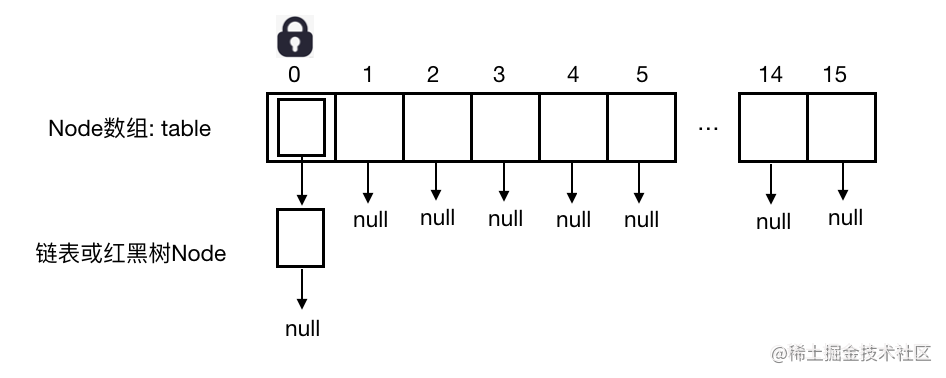Android

Android